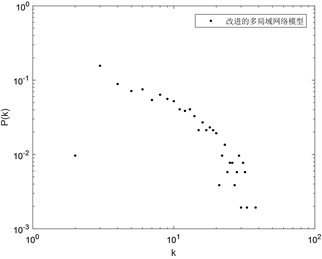# 一種改進的多局域世界網絡模型An Improved Multi-Local-World Network Model

• 全文下載: PDF(472KB)    PP.285-291   DOI: 10.12677/ORF.2019.94033
• 下載量: 124  瀏覽量: 815

On the basis of Multi-Local-World network model, this paper introduces the inequality and com-petitiveness among local networks, constructs an improved Multi-Local-World model, and analyses the degree distribution of the model. The network degree distribution of the model obeys power-law distribution, and the network is scale-free network.

1. 引言

2. 模型建立與分析

2.1. 改進的多局域世界模型(IMLW)

1) 以概率 ${p}_{1}$ 增加一個新節點到一個選定的局域網中，且與該局域網中的節點建立 ${n}_{1}$ 條邊。首先需要選定一個局域網Ωp，其概率為 $\frac{{r}_{p}}{\underset{q=1}{\overset{m}{\sum }}{r}_{q}}$ (2.11)，接下來新節點與該網絡節點i連接的概率為按照度擇優的原則(2.12)

2) 以概率 ${p}_{2}$ 增加 ${n}_{2}$ 條邊到選定的局域網中。首先需要選首先按照2.11式選定一個局域網 ${\Omega }_{p}$，隨機

3) 以概率 ${p}_{3}$ 在選定的局域網中去掉 ${n}_{3}$ 條邊。首先需要選定一個局域網 ${\Omega }_{p}$，根據反擇優原則，那么一個局域網的競爭力越低被選擇的概率就越大。選定局域網Ωp的概率為 $1?\frac{{r}_{p}}{\underset{q=1}{\overset{m}{\sum }}{r}_{q}}$，接下來隨機選擇一個節點，概率為 $\frac{1}{{N}_{{\Omega }_{p}}\left(t\right)}$，然后反擇優選擇另一節點，其概率為

${\prod }^{\prime }\left({k}_{i}\right)=\frac{1}{{N}_{{\Omega }_{p}}\left(t\right)?1}\left(1?\prod \left({k}_{i}\right)\right)=\frac{1}{{N}_{{\Omega }_{p}}\left(t\right)?1}\left(1?\frac{{k}_{i}}{\underset{j\in {\Omega }_{p}}{\sum }{k}_{j}}\right)$

4) 以概率 ${p}_{4}$ 選定一個局域網，讓其與其它局域網建立 ${n}_{4}$ 條長程邊。首先按照2.11選定一個局域網Ωr，然后在該網絡中按照2.12式選定一個節點，再按2.11式選擇一個局域網Ωp，并在該局域網中按2.12式選擇一個節點與之前節點相連，重復上述過程 ${n}_{4}$ 次。

5) 終止條件，運行N步。

2.2. 度分布分析

1) 增加一個帶有 ${n}_{1}$ 條邊的節點到一個選定的局域網Ωp中。

${\left(\frac{?{k}_{i}}{?t}\right)}_{1}=\frac{{n}_{1}{p}_{1}{r}_{p}}{\underset{q=1}{\overset{m}{\sum }}{r}_{q}}\frac{{k}_{i}}{\underset{j\in {\Omega }_{p}}{\sum }{k}_{j}}$ (2.13)

2) 增加 ${n}_{2}$ 條邊到選定的局域網中。

${\left(\frac{?{k}_{i}}{?t}\right)}_{2}=\frac{{n}_{2}{p}_{2}{r}_{p}}{\underset{q=1}{\overset{m}{\sum }}{r}_{q}}\left[\frac{1}{{N}_{{\Omega }_{p}}\left(t\right)}+\left(1?\frac{1}{{N}_{{\Omega }_{p}}\left(t\right)}\right)\frac{{k}_{i}}{\underset{j\in {\Omega }_{p}}{\sum }{k}_{j}}\right]$ (2.14)

3) 在選定的局域網中去掉 ${n}_{3}$ 條邊。

${\left(\frac{?{k}_{i}}{?t}\right)}_{3}=?{n}_{3}{p}_{3}\left(1?\frac{{r}_{p}}{\underset{q=1}{\overset{m}{\sum }}{r}_{q}}\right)\left[\frac{1}{{N}_{{\Omega }_{p}}\left(t\right)}+\left(1?\frac{1}{{N}_{{\Omega }_{p}}\left(t\right)}\right)\frac{1}{{N}_{{\Omega }_{p}}\left(t\right)?1}\left(1?\frac{{k}_{i}}{\underset{j\in {\Omega }_{p}}{\sum }{s}_{j}}\right)\right]$ (2.15)

4) 選定一個局域網，讓其與其它局域網建立 ${n}_{4}$ 條長程邊。

${\left(\frac{?{k}_{i}}{?t}\right)}_{4}={n}_{4}{p}_{4}\left(\frac{{r}_{p}}{\underset{q=1}{\overset{m}{\sum }}{r}_{q}}\frac{{k}_{i}}{\underset{j\in {\Omega }_{p}}{\sum }{k}_{j}}+\frac{{r}_{j}}{\underset{q=1}{\overset{m}{\sum }}{r}_{q}}\frac{{k}_{i}}{\underset{j\in {\Omega }_{p}}{\sum }{k}_{j}}\right)$ (2.16)

${N}_{{\Omega }_{p}}\left(t\right)={m}_{p}+\frac{{r}_{p}}{\underset{q=1}{\overset{m}{\sum }}{r}_{q}}{p}_{1}t$ (2.17)

$\begin{array}{c}\frac{?{k}_{i}}{?t}={\left(\frac{?{k}_{i}}{?t}\right)}_{1}+{\left(\frac{?{k}_{i}}{?t}\right)}_{2}+{\left(\frac{?{k}_{i}}{?t}\right)}_{3}+{\left(\frac{?{k}_{i}}{?t}\right)}_{4}\\ =\frac{{n}_{1}{p}_{1}{r}_{p}}{\underset{q=1}{\overset{m}{\sum }}{r}_{q}}\frac{{k}_{i}}{\underset{j\in {\Omega }_{p}}{\sum }{k}_{j}}+\frac{{n}_{2}{p}_{2}{r}_{p}}{\underset{q=1}{\overset{m}{\sum }}{r}_{q}}\left[\frac{1}{{N}_{{\Omega }_{p}}\left(t\right)}+\left(1?\frac{1}{{N}_{{\Omega }_{p}}\left(t\right)}\right)\frac{{k}_{i}}{\underset{j\in {\Omega }_{p}}{\sum }{k}_{j}}\right]\\ \text{?}\text{?}?{n}_{3}{p}_{3}\left(1?\frac{{r}_{p}}{\underset{q=1}{\overset{m}{\sum }}{r}_{q}}\right)\left[\frac{1}{{N}_{{\Omega }_{p}}\left(t\right)}+\left(1?\frac{1}{{N}_{{\Omega }_{p}}\left(t\right)}\right)\frac{1}{{N}_{{\Omega }_{p}}\left(t\right)?1}\left(1?\frac{{k}_{i}}{\underset{j\in {\Omega }_{p}}{\sum }{k}_{j}}\right)\right]\\ \text{?}\text{?}+{n}_{4}{p}_{4}\left[\frac{{r}_{p}}{\underset{q=1}{\overset{m}{\sum }}{r}_{q}}\frac{{k}_{i}}{\underset{j\in {\Omega }_{p}}{\sum }{k}_{j}}+\frac{{r}_{j}}{\underset{q=1}{\overset{m}{\sum }}{r}_{q}}\frac{{k}_{i}}{\underset{j\in {\Omega }_{p}}{\sum }{k}_{j}}\right]\end{array}$

$\begin{array}{l}=\frac{{n}_{1}{p}_{1}}{c}?\frac{{k}_{i}}{t}+\frac{{n}_{2}{p}_{2}}{{p}_{1}}?\frac{1}{t}+\frac{{n}_{2}{p}_{2}}{c}?\frac{{k}_{i}}{t}?{n}_{2}{p}_{2}?\frac{\underset{q=1}{\overset{m}{\sum }}{r}_{q}}{{r}_{p}c{p}_{1}}?\frac{{k}_{i}}{{t}^{2}}+\frac{{n}_{3}{p}_{3}}{{p}_{1}}?\frac{1}{t}?{n}_{3}{p}_{3}?\frac{\underset{q=1}{\overset{m}{\sum }}{r}_{q}}{{r}_{p}{p}_{1}}?\frac{1}{t}\\ \text{?}\text{?}+{n}_{3}{p}_{3}?\frac{\underset{q=1}{\overset{m}{\sum }}{r}_{q}?{r}_{p}}{\underset{q=1}{\overset{m}{\sum }}{r}_{q}}+{n}_{3}{p}_{3}?\frac{\underset{q=1}{\overset{m}{\sum }}{r}_{q}?{r}_{p}}{c{r}_{p}}\frac{{k}_{i}}{t}+{n}_{3}{p}_{3}?\frac{\left(\underset{q=1}{\overset{m}{\sum }}{r}_{q}?{r}_{p}\right)?\underset{q=1}{\overset{m}{\sum }}{r}_{q}}{{p}_{1}{r}_{p}t\left({p}_{1}{r}_{p}t?\underset{q=1}{\overset{m}{\sum }}{r}_{q}\right)}\\ \text{?}\text{?}+{n}_{3}{p}_{3}?\frac{\left(\underset{q=1}{\overset{m}{\sum }}{r}_{q}?{r}_{p}\right)?{\left(\underset{q=1}{\overset{m}{\sum }}{r}_{q}\right)}^{2}}{c{p}_{1}{r}_{p}t\left({p}_{1}{r}_{p}t?\underset{q=1}{\overset{m}{\sum }}{r}_{q}\right)}?\frac{{k}_{i}}{t}+\frac{2{n}_{4}{p}_{4}}{c}?\frac{{k}_{i}}{t}\\ =\frac{{n}_{1}{p}_{1}}{c}?\frac{{k}_{i}}{t}+\frac{{n}_{2}{p}_{2}}{c}?\frac{{k}_{i}}{t}+{n}_{3}{p}_{3}?\frac{\underset{q=1}{\overset{m}{\sum }}{r}_{q}?{r}_{p}}{c{r}_{p}}\frac{{k}_{i}}{t}+\frac{2{n}_{4}{p}_{4}}{c}?\frac{{k}_{i}}{t}\\ \text{?}\text{?}+\frac{{n}_{2}{p}_{2}}{{p}_{1}}?\frac{1}{t}+\frac{{n}_{3}{p}_{3}}{{p}_{1}}?\frac{1}{t}?{n}_{3}{p}_{3}?\frac{\underset{q=1}{\overset{m}{\sum }}{r}_{q}}{{r}_{p}{p}_{1}}?\frac{1}{t}\end{array}$ (2.20)

$a=\frac{{n}_{1}{p}_{1}}{c}+\frac{{n}_{2}{p}_{2}}{c}+\frac{\underset{q=1}{\overset{m}{\sum }}{r}_{q}?{r}_{p}}{c{r}_{p}}+\frac{2{n}_{4}{p}_{4}}{c}$$b=\frac{{n}_{2}{p}_{2}}{{p}_{1}}+\frac{{n}_{3}{p}_{3}}{{p}_{1}}?{n}_{3}{p}_{3}?\frac{\underset{q=1}{\overset{m}{\sum }}{r}_{q}}{{r}_{p}{p}_{1}}$

$\frac{?{k}_{i}}{?t}=a?\frac{{k}_{i}}{t}+b?\frac{1}{t}$

${k}_{i}\left(t\right)=\underset{{t}_{i}}{\overset{t}{\int }}a?\frac{{k}_{i}}{t}+b?\frac{1}{t}\text{d}t=\left({n}_{1}+\frac{b}{a}\right){\left(\frac{t}{{t}_{i}}\right)}^{a}?\frac{a}{b}$

$\begin{array}{c}F\left(k\right)=P\left({k}_{i}\left(t\right){\left(k+\frac{b}{a}\right)}^{?\frac{1}{a}}{\left({n}_{1}+\frac{b}{a}\right)}^{\frac{1}{a}}t\right)\\ =1?\frac{t}{M+t}{\left(s+\frac{b}{a}\right)}^{?\left(\frac{1}{a}+1\right)}{\left({n}_{1}+\frac{b}{a}\right)}^{\frac{1}{a}}\end{array}$

$\frac{1}{a}=\frac{1}{\frac{{n}_{1}{p}_{1}}{c}+\frac{{n}_{2}{p}_{2}}{c}+\frac{\underset{q=1}{\overset{m}{\sum }}{r}_{q}?{r}_{p}}{c{r}_{p}}+\frac{2{n}_{4}{p}_{4}}{c}}=\frac{2\left({n}_{1}{p}_{1}+{n}_{2}{p}_{2}?{n}_{3}{p}_{3}+2{n}_{4}{p}_{4}\right){r}_{p}}{{n}_{1}{p}_{1}{r}_{p}+{n}_{2}{p}_{2}{r}_{p}+\underset{q=1}{\overset{m}{\sum }}{r}_{q}?{r}_{p}+2{n}_{4}{p}_{4}{r}_{p}}$${r}_{p}\left(p=1,2,?,m\right)$ 的取值影響著冪指數的值，當 ${r}_{p}\left(p=1,2,?,m\right)$ 取值均相同時，冪指數的值介于1~2之間。

3. 仿真模擬Figure 1. MLW degree distributionFigure 2. IMLW degree distribution

4. 小結

  Chen, G., Fan, Z.P., Li, X. (2005) Modeling the complex Internet topology. In: Vattay, G. and Ocarev, L.K, Eds., Complex Dynamics in Communication Network, Springer-Verlag, Berlin.  田思, 李慧嘉, 趙岳. 一種新型多局域世界網絡模型分析[J]. 計算機應用研究, 2013, 30(3): 869-872.  李曉. 基于雙向擇優機制的多局域加權網絡研究[D]: [碩士學位論文]. 濟南: 山東師范大學, 2012.  汪小帆. 復雜網絡理論及其應用[M]. 北京: 清華大學出版社, 2005.